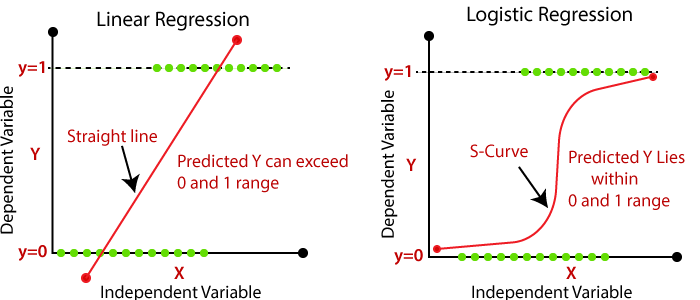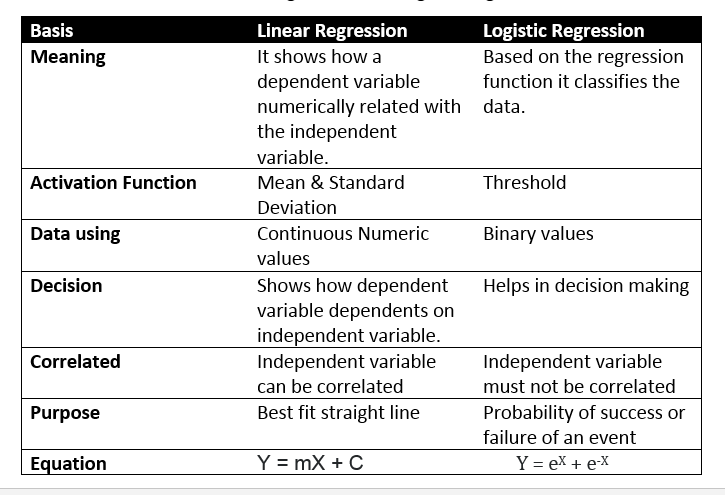By- Vineet Maheshwari
To infinity and beyond.

0 likes followers ViewsVineet Maheshwari

Followers
Views
0 Likes

# Logistic Regression

TechnologyIn the previous article, we have seen that there are various regression techniques and we also learnt about Linear Regression Model, so now is the time to learn about Logistic Regression.

Like every other regression technique, Logistic Regression is also a technique for predictive analysis. It is used for establishing a relationship between one dependent and one or more independent variable. It is applicable when the dependent variable is categorical.

Logistic regression is not much different from linear regression, except that a Sigmoid function is being fit into the equation of linear regression.

Linear regression equation :

y = a + b1x + b2x + b3x + ....

Sigmoid function :

p = 1 / (1 + e ^ (-y))

Therefore it becomes,

p = 1 / (1 + e ^ (-a -b1x -b2x -...))So this is the basic difference between these two techniques, addition of sigmoid function changes everything.

Types of Logistic Regression :

1. Binary Logistic Regression : There are only two possible outcomes, such as Yes or No, Spam or no Spam. These are the outcomes of target variable.
2. Multinomial Logistic Regression : The target variable has three or more nominal categories and that too, without ordering, such as predicting that which superstar is more preferred, which food is more preferred, predicting the type of wine.
3. Ordinal Logistic Regression : The target variable has three or more ordinal categories, it means the categories are in order. For example, rating any product, restaurant, movie from 1 to 5.

Now how is this technique different from other techniques ?

Well quite an interesting question, isn’t it ? We have got an answer to this question as well.

The essential difference between logistic and linear regression is, Logistic is being used when the dependent variable is binary in nature in contrast to Linear regression, where the dependent variable is continuous and the regression line is also linear. In logistic regression, sigmoidal function is being used along with the equation linear regression equation. Moreover, the independent variables could be correlated with each other in Linear regression, but in logistic regression it is more preferable if no correlation exist between the independent variables.Implementation in python :

There are some in-built libraries that help us to do our work easily and most of our work is being done by them only.

#sklearn is the required library

import numpy as np

import matplotlib.pyplot as plt

from sklearn.linear_model import LogisticRegression

from sklearn import datasets

iris = dataset.load_iris() #loading our data

x = iris.data[:, :2] #selecting the first two features

y = iris.target

logreg = LogisticRegression()

logreg.fit(x,y)

logreg.predict(iris.data[-1,:],iris.target[-1])

#output is this and it may change

#and it is for three classes 0,1,2

#[0.28, 0.71, 0.016]

Applications of Logistic regression :

1. This is a very useful technique in the field of Marketing, for predicting if the company will make profit, loss or it will remain ate break-even based on the operations.
2. It can be used by the company to predict the attendance of their employees by studying the pattern in which they take leaves, and also according to their individual characteristics.
3. Can turn out to be a useful technique for medical purposes. It can predict the medical condition of a patient based on hi/her medical history, symptoms and individual characteristics and also comparing him/her with other patients as well.
4. Because of it’s efficient and straight-forward nature, it is easy to implement and therefore it is widely used by data analyst and scientist.

Assumptions :

Now with the whole functioning of every technique, there are some assumptions as well that we have to take care of :

1. The error terms are NOT to be normally distributed.
2. It does NOT require linear relationship between dependent and independent variables.
3. Dependent variables are not measured on a ratio scale.
4. Dependent variable must be categorical.
5. There should be a little or no correlation between independent variables.

There are some disadvantages as well :

1. It can not handle large number of categorical variables, it means it cannot be used on a heavy model.
2. Major drawback is, it is vulnerable to overfitting.
3. If independent variables are not correlated with the target variable, then this technique does not work properly.

HelpFeaturesMade with in INDPrivacyAbout
© 2020 Peppychunk.com